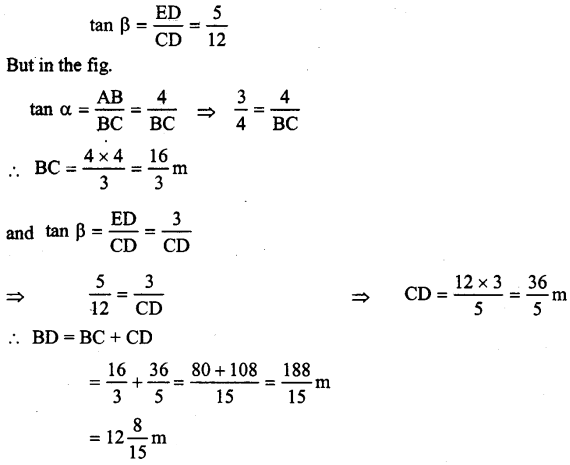## ML Aggarwal Class 9 Solutions for ICSE Maths Chapter 17 Trigonometric Ratios Chapter Test

Question 1.
(a) From the figure (i) given below, calculate all the six t-ratio for both acute angles.
(b) From the figure (ii) given below, find the values of x and y in terms of t-ratio of θ.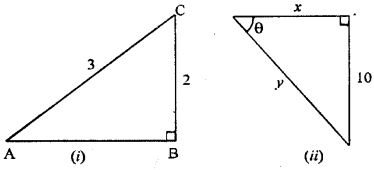Given ∆ ABC is right angled at B,
Then in right angled ∆ ABC,
By Pythagoras theorem, we get
AC2 = AB2 + BC2
⇒ AB2 = AC2 – BC2
⇒ AB2 = (3)2 – (2)2
⇒ AB2 = 9 – 4
⇒ AB2 = 5
AB = $$\sqrt{{5}}$$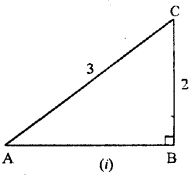(b) Let ABC be given triangle in which B is at right angle and ∠ BAC = θ
Then we know that,Question 2.
(a) From the figure (1) given below, find the values of:
(i) sin ∠ ABC
(ii) tanx – cosx + 3sinx.
(b) From the figure (2) given below, find the values of;
(i) 5 sin x
(ii) 7 tan x
(iii) 5 cos x – 17 siny – tan x.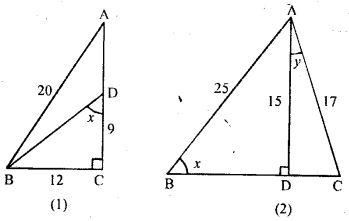(a) In this figure
BC = 12, CD = 9 and BC = 20
In right angled ∆ ABC,By Pythagoras theorem, we get
AB2 = AC2 + BC2
2 = AB2 – BC2
⇒ AC2 = (20)2 – (12)2
⇒ AC2 = 400 – 144
⇒ AC2 = 256 ⇒ AC2 = (16)2 ⇒ AC = 16
But, In right angled ∆ BCD,
By Pythagoras theorem, we get
BD2 = 2 + CD2
⇒ BD2 = (12)2 + (9)2
BD2= 144 + 81 ⇒ BD2 = 225 ⇒ BD2 = (15)2
⇒ BD = 15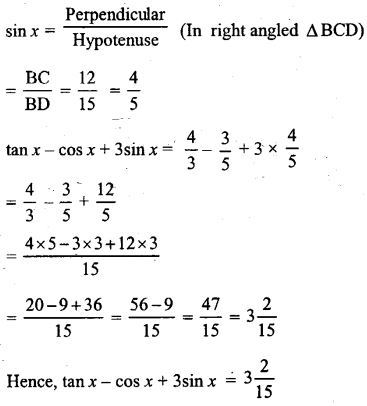(b) From figure AC = 17, AB = 25, AD = 15
In right angled ∆ ACD By Pythagoras theorem, we getAC2 = AD2 + CD(17)2 = (15)2 + (CD)2
⇒ CD2 = (17)2 – (15)2
⇒ CD2 = 289 – 225
⇒ CD2 = 64
⇒ CD2 = (8)2
⇒ CD = 8
In right angled ∆ABD,
By Pythagoras theorem, we get ,
⇒ (25)2 = (15)2 + BD2 ⇒ BD2 = (25)2 – (15)2
⇒ BD2 = 625 – 225 ⇒ BD2 = 400
⇒ BD2 = (20)2 ⇒ BD = 20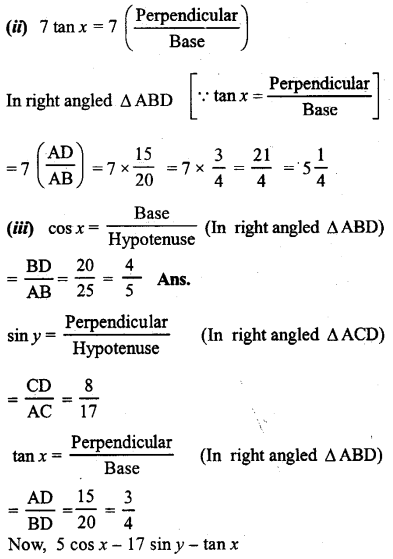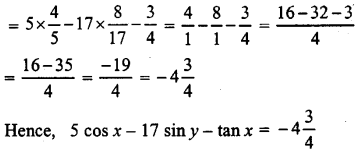Question 3.
If q cosθ = p, find tan θ – cot θ in terms of p and q.
Let ABC be a right angled triangle and B at right angled and ∠ ACB = 0 .Given that,
q cos θ = p ⇒ cos θ = $$\frac{p}{q}$$
⇒ $$\frac{\mathrm{BC}}{\mathrm{AC}}=\frac{p}{q}$$
Let BC = px then AC = qx
In right angled ∆ ABC,
By Pythagoras theorem, we get
AC2 = AB2 + BC2
⇒ AB2 = AC2 – BC2
⇒ AB2 = (qx)2 – (px)2
⇒ AB2 = q2x2 – p2x2
⇒ AB2 = (q2 – p2) x2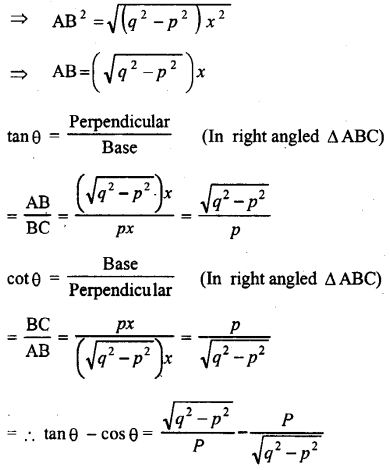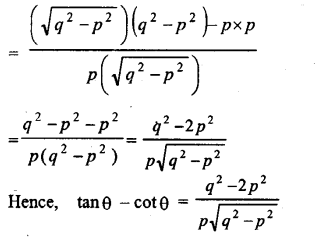Question 4.
Given 4 sin θ =3 cos θ, find the values of :
(i) sin θ
(ii) cos θ
(iii) cot2 θ – cosec2 θ.
Given that, 4sin θ = 3cosθ
⇒ $$\frac{\sin \theta}{\cos \theta}=\frac{3}{4}$$Question 5.
If 2 cos θ = $$\sqrt{{3}}$$, prove that 3 sin θ – 4 sin3 θ = 1
2 cos θ = $$\sqrt{{3}}$$Hence proved.

Question 6.
If $$\frac{\sec \theta-\tan \theta}{\sec \theta+\tan \theta}=\frac{1}{4}, \text { find } \sin \theta$$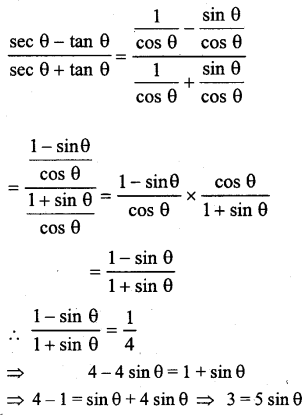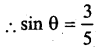Question 7.
If sin θ + cosec θ = $$3 \frac{1}{3}$$, find the value of sin2 θ + cosec2 θ.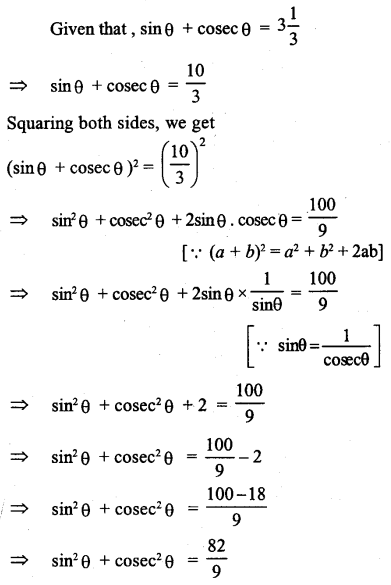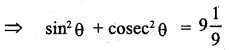Question 8.
In the adjoining figure, AB = 4 m and ED = 3 m.
If sin α = $$\frac{3}{5}$$ and cos β = $$\frac{12}{13}$$ , And the length of BD.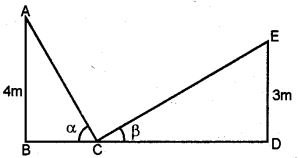sin α = $$\frac{3}{5}$$ = $$\frac{AB}{AC}$$
∴ AB = 3, AC = 5
But AC2 = AB2 + BC2
⇒ (5)2 = (3)2 + BC2 ⇒ 25 = 9 + BC2
⇒ BC2 = 25 – 9 = 16 = (4)-2 ⇒ BC = 4
∴ tan α = $$\frac{AB}{BC}$$ = $$\frac{4}{5}$$
cos β = $$\frac{12}{13}$$ = $$\frac{CD}{CE}$$
∴ CD = 12, CE = 13
But CE2 = CD2 + ED2
(13)2 = (12)2 + (ED)2
⇒ 169 = 144 + ED2 ⇒ ED2 = 169 – 144 = 25 = (5)2
∴ ED = 5